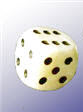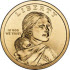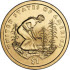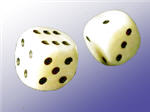# 随机变量

### 例子：抛硬币：结果可以是正面或反面。X = {0, 1}

• 有个实验（比方抛硬币）
• 我们给每个事件分派数值
• 数值的集合是个随机变量

## 不像代数变量

### 例子：X = {0, 1, 2, 3}

X 可以随机地取 0、1、2、或 3。

## 样本空间### 例子：掷一次骰子

X 可以是 1、2、3、4、5 或 6

## 概率

P(X = 值) = 值的概率

### 例子（续）：掷一次骰子

X = {1, 2, 3, 4, 5, 6}

• P(X = 1) = 1/6
• P(X = 2) = 1/6
• P(X = 3) = 1/6
• P(X = 4) = 1/6
• P(X = 5) = 1/6
• P(X = 6) = 1/6### 例子：抛 3个硬币会得到几个正面？

X = "正面的个数" 是随机变量。

 X = "正面的个数" HHH3 HHT2 HTH2 HTT1 THH2 THT1 TTH1 TTT0

• P(X = 3) = 1/8
• P(X = 2) = 3/8
• P(X = 1) = 3/8
• P(X = 0) = 1/8### 例子：掷两个骰子

第一个骰子 1 2 3 4 5 2 3 4 5 6 7 3 4 5 6 7 8 4 5 6 7 8 9 5 6 7 8 9 10 6 7 8 9 10 11 7 8 9 10 11 12

• 2 只出现一次，所以 P(X = 2) = 1/36
• 3 出现两次，所以 P(X = 3) = 2/36 = 1/18
• 4 出现三次，所以 P(X = 4) = 3/36 = 1/12
• 5 出现四次，所以 P(X = 5) = 4/36 = 1/9
• 6 出现五次，所以 P(X = 6) = 5/36
• 7 出现六次，所以 P(X = 7) = 6/36 = 1/6
• 8 出现五次，所以 P(X = 8) = 5/36
• 9 出现四次，所以 P(X = 9) = 4/36 = 1/9
• 10 出现三次，所以 P(X = 10) = 3/36 = 1/12
• 11 出现两次，所以 P(X = 11) = 2/36 = 1/18
• 12 出现一次，所以 P(X = 12) = 1/36

## 一个范围内的值

### 例子（续） 两个骰子的点数的和是 5、6、7 或 8 的概率是多少？

P(5 X ≤ 8) = P(X = 5) + P(X = 6) + P(X = 7) + P(X = 8) = (4+5+6+5)/36 = 20/36 = 5/9

## 解法

### 例子（续） 如果 P(X = x) = 1/12，x 的值是多少？

P(X = 4) = 1/12，P(X = 10) = 1/12

• X 是随机变量 "两个骰子点数的和"。
• xX 的可能值。

## 连续

• 离散数据只能取某些数值（例如 1、2、3、4、5）
• 连续数据可以取一个范围（值域）里的任何数值（例如人的身高）

## 总结

• 随机变量是随机实验结果的可能数值的集合。
• 可能数值的集合是它的样本空间
• 随机变量使用大写字母来代表，例如 X 或 Z。
• 随机变量可以是离散或连续的。